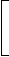# Data Interpretation - Pie Charts - Discussion

Study the following pie-diagrams carefully and answer the questions given below it:

Percentage Composition of Human Body1.

What percent of the total weight of human body is equivalent to the weight of the proteins in skin in human body?

 [A]. 0.016 [B]. 1.6 [C]. 0.16 [D]. Data inadequate

Explanation:

Let the body weight be x kg.

Then, weight of skin protein in the body
 =16% of1 of xkg 10
 =16 xkg. 1000Required percentage =16x1000
x 100% = 1.6%.
x

 Jayati said: (Aug 13, 2012) Both the compositions are given for the entire body. How can we find the composition in an individual component. The answer should be data inadequate.

 Aman Jain said: (Jul 25, 2014) The answer should be data inadequate as the fraction of proteins in boned has not been provides in the question.

 Kasinath @Hyd said: (Aug 21, 2014) Proteins, 16% in 100% = 0.16%. Skin 1/10*100= 10%. Proteins in skin= 0.16% * 10% =1.6%.

 Kasinath @Hyd said: (Aug 21, 2014) Wt of proteins = 16%. Wt of Skin = 1/10. converting in to %value => 1/10*100 = 10%. Wt of proteins in skin = 16% * 10%. Human body Wt = 100%. =>(16*10)/100 = 1.6%.

 David said: (Oct 3, 2014) Put most simply: 1/10 = 0.1. 16% of 0.1 = (0.1 * 0.16) = 0.016. As a percentage: 0.016 * 100 = 1.6%.

 Trishul said: (Jul 19, 2015) Assuming that water, proteins and other dry elements are uniformly distributed across various body parts given in the 1st pie chart. It should be mentioned in the question.

 Vicky said: (Aug 20, 2016) X% = 16% * 1/10. X/100 = (16/100) * (1/10). X = 16/10. X = 1.6.

 Deepshikha Sarda said: (Jun 9, 2017) Yes, I agree @Trishul.

 Sushmitha said: (Apr 28, 2018) How 1000 came? Please explain.

 Victor said: (Dec 27, 2019) Assuming that who make this question have 70% water in his brain.